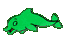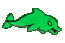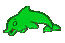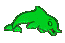Probability of Independent and Dependent Events     Math Help     Game Tips:

- A 50 percent chance of rain tomorrow means the probability of rain tomorrow is 0.5 or 1/2.
Probability can be represented by a percent, decimal or fraction.

- In general for equally likely events, a probability P equals the number of favourable outcomes
divided by the total number of possible outcomes.

- If your name is entered 20 times in a random contest with 80 other names, then the chance of
your name being the one name chosen from the 100 equally likely names is 20/100 = 0.2 = 20%.

- If your name is entered 20 times in a random contest with 80 other names, then the chance of your
name being chosen twice in a row is (20/100)(19/99) = 380/9900 = approx 3.8%.
In this case, the two name draws are dependent events since the first favourable name drawn
is missing for the second draw and affects the outcome of the second draw.

- If your name is entered 20 times in a random contest with 80 other names, then the chance of your
name being chosen twice in a row with name replacement is (20/100)(20/100) = 400/10000 = 4.0%.
In this case, the two name draws are independent events since
the first favourable name drawn is re-entered for the second draw.

- In this game, if a chosen object is to be replaced, the question will mention the replacement.
If replacement is not mentioned in a question, then assume the chosen object was not replaced.

- In general, two events are independent when the outcome of the first does not affect the second.

- In general when 2 events are independent, the probability of the 2 events occurring equals
P = (the probability of the first event) multiplied by (the probability of the second event).

- Your Game Score is reduced by the number of dolphin hits.

- To slow the game speed repeat tap/click on the word Slider.
- To increase the game speed repeat tap/click on the word Math.
- Speed can also be adjusted with a keyboard's - and + keys.

- Refresh/Reload the web page to restart the game.